# Animation of Fractal Objects

## www.lactamme.polytechnique.fr

#### CMAP (Centre de Mathématiques APpliquées) UMR CNRS 7641, École polytechnique, Institut Polytechnique de Paris, CNRS, France france telecom, France Telecom R&D

[Site Map, Help and Search [Plan du Site, Aide et Recherche]]
[The Y2K Bug [Le bug de l'an 2000]]
[Real Numbers don't exist in Computers and Floating Point Computations aren't safe. [Les Nombres Réels n'existent dans les Ordinateurs et les Calculs Flottants ne sont pas sûrs.]]
[Please, visit A Virtual Machine for Exploring Space-Time and Beyond, the place where you can find thousands of pictures and animations between Art and Science]
(CMAP28 WWW site: this page was created on 07/16/1997 and last updated on 11/14/2023 17:46:14 -CET-)

(published in Computer Graphics Interface'89, Canada, 06/1989)

Abstract: This paper describes a new method for the generation of fractal objects like mountains and clouds. It is based on the superposition of independent N-dimensional meshes. It is shown that with meshes of dimension higher than 2, it allows the animation of fractal objects, and for example the simulation of cloud dynamics and earthquakes.

Keywords: animation, clouds, cloud dynamics, earthquakes, fractal objects, mountains.

Contents:

# 1-INTRODUCTION:

## 1.1-The recursive subdivision algorithm:

To generate, for example, mountain surfaces using fractal techniques, they are well known methods like Fourier transform of white noise and recursive subdivision . The first one is slow, and the second one shows artifacts. Let's recall it. This algorithm starts with a triangle or a quadrilateral: for this last case, let {V(1),V(2),V(3),V(4)} be the initial two-dimensional quadrilateral; on each side {V(i),V(i+1)} let's choose (deterministically or randomly) a point P(i,i+1) and inside of {V(1),V(2),V(3),V(4)} a fifth point P. Then the five points P, P(1,2), P(2,3), P(3,4) and P(4,1) are randomly moved along the third dimension, thus giving birth to four new "three-dimensional" quadrilaterals:

• {V(1),P(1,2),P,P(4,1),V(1)},
• {P(1,2),V(2),P(2,3),P,P(1,2)},
• {P(2,3),V(3),P(3,4),P,P(2,3)},
• {P(3,4),V(4),P(4,1),P,P(3,4)}.

Then the process is iterated recursively for each new quadrilateral; at the end, we obtain an irregular surface. This process frequently shows artefacts (like creases of slope discontinuities ) and cannot model easily smooth terrains.

## 1.2-The superposition of independent two-dimensional meshes:

We propose a new method based on the superposition of independent N-dimensional meshes. To be clear, let's describe it with a two-dimensional example. We shall use a set of square meshes {M(1),M(2),...,M(m)} of decreasing sizes (the smallest one is on the order of the pixel size). We never require coincidences between nodes belonging to various meshes, unlike the recursive subdivision algorithm; thus the computations of all the meshes will be independent. For the mesh M(k) (k [1,m]) we generate on each vertex V(i,j) a random value RDN(i,j,k,S) as a function of i and j (the coordinates relating to the mesh M(k)), k (the rank of the mesh) and S (a seed for the random generator). Then the mesh is "rasterized" in the following way: if the current point P(x,y) is a vertex V(i,j), it will receive the value RDN(i,j,k,S), otherwise, its value will be interpolated between the values of its neighbours. Finally, a two-dimensional field is obtained by superposing and adding point by point all the rasterized meshes; figures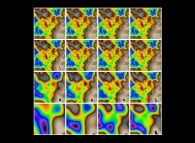,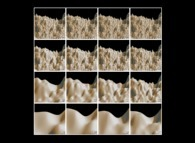and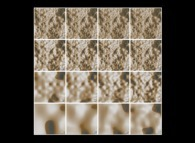show the sixteen first steps of this iterative process. Then this field can be visualized as a two-dimensional field, as a three-dimensional surface (the value at the point P(x,y) giving the third coordinate), or again transformed (see figure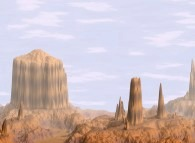) and combined with other two-dimensional fields (see figures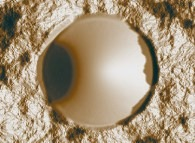and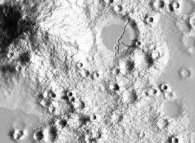). The appendix gives a view of the available tools for the manipulation of two-dimensional fields.

With this two-dimensional example, it may be seen that this method gives us four "degrees of freedom":

• the geometry of the meshes,
• the interpolation function,
• the random generator,
• and finally the mapping function between meshes and space coordinates.

Two interpolation functions have been implemented: bi-linear and bi-cubic. When used with meshes such that:
```                                  size(M(k-1))
size(M(k)) = --------------
2
```
and such that one vertex of M(k) coincide with one vertex of M(k-1), the bi-linear interpolation can simulate the subdivision algorithm; but unfortunately, this method (the fastest) shows clearly slope discontinuities, when the bi-cubic one looks more realistic.

# 2-THE SUPERPOSITION OF INDEPENDENT n-DIMENSIONAL MESHES:

## 2.1-Definitions:

Let E be a subset of R^n with coordinates {x(1),x(2),...,x(n)}. Let {M(1),M(2),...,M(k),...,M(m)} be a family of hypercellular meshes of decreasing sizes:
```                    size(M(1)) > size(M(2)) >...> size(M(k)) >...> size(M(m)).
```
It is important to notice that this family is not obtained with a recursive subdivision and that all its elements are independent. Let RDN(x(1),x(2),...,x(n),k,S) be a random generator; its arguments are the coordinates {x(1),x(2),...,x(n)}, the rank k of the current mesh M(k) and a seed S.

## 2.2-The hyperfield:

For each point (x(1),x(2),...,x(n)) of E and for each mesh M(k) we define a scalar hyperfield Fk:
```                    Fk(x(1),x(2),...,x(n),k,S) = RDN(x(1),x(2),...,x(n),k,S)
```
if the point {x(1),x(2),...,x(n)} of E is a vertex of the current mesh M(k), and:
```                    Fk(x(1),x(2),...,x(n),k,S) = Interpolation(RDN(X(1),X(2),...,X(n),k,S))
```
computed for all adjoining vertices {X(1),X(2),...,X(n)} otherwise.

Here again, the Interpolation function is an arbitrary one: it can be a n-linear one using the 2^n nearest nodes, a n-cubic one, or again, any other function. This is just another parameter...

## 2.3-The N-dimensional fractal field:

For each point }x(1),x(2),...,x(n)} of E we define a N-dimensional fractal field F:
```                                               k=m
-----
\
F(x(1),x(2),...,x(n),S) = /     p(k).Fk(x(1),x(2),...,x(n),k,S)
-----
k=1
```
where p(k) is a ponderation factor such that:
```                    p(1) > p(2) >...> p(k) >...>  p(m).
```
The way p(k) is defined is arbitrary, but can be chosen, for example, proportional to the volume of the elementary cell of the mesh M(k).

# 3-ANIMATION OF FRACTAL OBJECTS:

Let S be the physical space; it is a subset of R^4 (or R^3, according to the dimension of the simulation) with coordinates (x,y,[z,]t). With these definitions, let's give two examples of the animation of fractal objects.

## 3.1-Earthquakes:

It suffice to use the preceding model with the following mapping between S (= R^3) and E:
```                    x -->  x(1),
y -->  x(2),
t -->  x(3).
```
Figure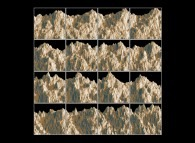shows sixteen frames from a terrific (because of the amplitude...) earthquake simulation. Each of the "instaneous" mountains is obtained with a two-dimensional cross-section inside the three-dimensional fractal field at t=x(3)=constant; then this "sub-field" is visualized as a three-dimensional surface.

## 3.2-Cloud dynamics:

It suffice to use the preceding model with the following mapping between S (= R^3 to reduce the computing time) and E:
```                    x --> [x(1) + dx(1,x(3))]
modulo L
x

y --> [x(2) + dx(2,x(3))]
modulo L
y

t --> [x(3)]
modulo L
t
```
where the vector {dx(1,x(3)),dx(2,x(3))} is used to simulate the effect of the wind in the (x,y) plane, and the modulo operators are here to allow the generation of periodic sequences. Figure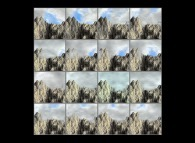shows us a complex scene: a fractal mountain obtained by the preceding method of superposition, with three two-dimensional fields of clouds with a wind blowing from the right to the left:
```                    dx(1,x(3)) = -PositiveValue.x(3),
dx(2,x(3)) = 0.
```
This simulation was only three-dimensional in order to reduce the computing time. About the shadows, the ones relating to the mountains are correct, when the ones relating to the clouds are simulated (due to their two-dimensionality...) with a texture mapping approach: at time t, a texture using the clouds at time t+Dt (Dt << Lt) is computed and then mapped onto the mountain surface.

# 4-CONCLUSION:

this method is general; it allows the generation of N-dimensional fractal objects, where one of the dimension can be the time, thus giving animation capabilities.

# APPENDIX:

This algorithm is in fact a small component of a much more larger software written to facilitate the manipulation and the visualization of large sets of scientific data . A set of cpp macros (giving birth to C or Fortran sources) is the programming language and UNIX, the operating sytem. Computing fractal objects as fields allows us to manipulate them with general tools. They are C-like functions, like mountain(parameters), and each has its counterpart at the Shell level: hundreds of "pipeable" commands are available. Thus it is valid to write (where 'parameters' denotes some lists of parameters):
```                    display(mountain(fractal_nD(parameters),parameters),parameters);
```
or again, as a string of piped commands:
```                    fractal_nD parameters | mountain parameters | display parameters
```
In both case, a N-dimensional fractal field is computed and displayed as a three-dimensional surface.